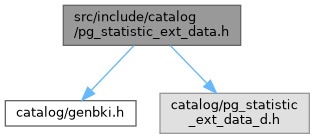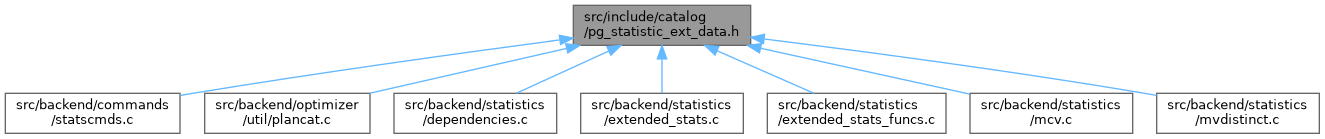PostgreSQL Source Code  git master
pg_statistic_ext_data.h File Reference
`#include "catalog/genbki.h"`
`#include "catalog/pg_statistic_ext_data_d.h"`
Include dependency graph for pg_statistic_ext_data.h:This graph shows which files directly or indirectly include this file:Go to the source code of this file.

## Typedefs

typedef FormData_pg_statistic_ext_dataForm_pg_statistic_ext_data

## Functions

CATALOG (pg_statistic_ext_data, 3429, StatisticExtDataRelationId)

## Variables

FormData_pg_statistic_ext_data

## ◆ Form_pg_statistic_ext_data

Definition at line 50 of file pg_statistic_ext_data.h.

## ◆ CATALOG()

 CATALOG ( pg_statistic_ext_data , 3429 , StatisticExtDataRelationId )

Definition at line 31 of file pg_statistic_ext_data.h.

32 {
33  Oid stxoid; /* statistics object this data is for */
34
35 #ifdef CATALOG_VARLEN /* variable-length fields start here */
36
37  pg_ndistinct stxdndistinct; /* ndistinct coefficients (serialized) */
38  pg_dependencies stxddependencies; /* dependencies (serialized) */
39  pg_mcv_list stxdmcv; /* MCV (serialized) */
40
41 #endif
42
unsigned int Oid
Definition: postgres_ext.h:31
FormData_pg_statistic_ext_data

## ◆ FormData_pg_statistic_ext_data

 FormData_pg_statistic_ext_data

Definition at line 43 of file pg_statistic_ext_data.h.